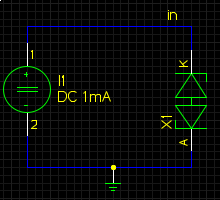# bidirectional zener diode test

## test schematic setupThere are only two test simulations for a zener diode: the forward voltage the reverse voltage Both simulations are done at different temperatures and plotted in two semilogy plots.

## postprocessing tests

The expected range for the forward and the reverse voltage should be between 0 and 200 volts. It will catch all model errors where either the reverse voltage is not specified or the simulator takes a standard diode because of various model errors.

## example test resultsforward current as a function of forward voltage at different temperatures.Reverse current as a function of reverse voltage at different temperatures.

Werner Hoch